Courses

# Test: Balancing - 2

## 10 Questions MCQ Test Topicwise Question Bank for Mechanical Engineering | Test: Balancing - 2

Description
This mock test of Test: Balancing - 2 for Mechanical Engineering helps you for every Mechanical Engineering entrance exam. This contains 10 Multiple Choice Questions for Mechanical Engineering Test: Balancing - 2 (mcq) to study with solutions a complete question bank. The solved questions answers in this Test: Balancing - 2 quiz give you a good mix of easy questions and tough questions. Mechanical Engineering students definitely take this Test: Balancing - 2 exercise for a better result in the exam. You can find other Test: Balancing - 2 extra questions, long questions & short questions for Mechanical Engineering on EduRev as well by searching above.
QUESTION: 1

### The maximum value of secondary force as compared to the maximum value of primary force is

Solution: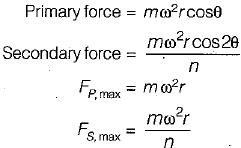QUESTION: 2

### The frequency of primary and secondary forces in reciprocating masses conform to the ratio

Solution:

Secondary force has twice the frequency of primary force is generally neglected at low speeds. Secondary force has twice the frequency of primary force is generally neglected at low speeds.

QUESTION: 3

### Which of the following parameter(s) must be balanced for complete balancing of reciprocating masses?

Solution:
QUESTION: 4

The partial balancing of primary forces in locomotives is generally of the order of

Solution:

In reciprocating masses, usually two-third of primary forces are balanced.

QUESTION: 5

In locomotive operation, the swaying couple is caused by

Solution:
QUESTION: 6

The partial balancing means

Solution:

Since reciprocating mass cannot balance fully hence partial balancing of reciprocating mass is done while revolving masses can be balanced fully.

QUESTION: 7

The maximum magnitude of the unbalanced force along the line perpendicular to the line of stroke is called

Solution:

Hammer Blow: The maximum magnitude of unbalanced force along the line perpendicular to the line of stroke is called hammer blow.

QUESTION: 8

The maximum magnitude of the unbalance force along the line parallel to the line of stroke is called

Solution:

Swaying couple: The maximum magnitude of the unbalanced force along the line parallel to the line of stroke is called swaying couple.

QUESTION: 9

The maximum and minimum value of tractive force is given by

Solution:

Tractive effort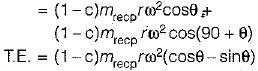For maximum and minimum tractive effort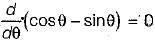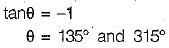Hence maximum and minimum value of tractive effort is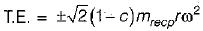QUESTION: 10

In case of partial balancing of single cylinder reciprocating engine what is the primary disturbing force along the line of stroke
where,
c = fraction of reciprocating mass to be balanced
ω - angular velocity of crank shaft
θ - crank angle

Solution: